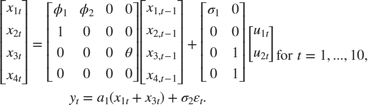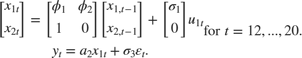Implicitly Create Time-Varying State-Space Model

This example shows how to create a time-varying, state-space model by passing a parameter-mapping function describing the model to ssm (i.e., implicitly create a state-space model).

Suppose that from periods 1 through 10, the state model are stationary AR(2) and MA(1) models, respectively, and the observation model is the sum of the two states. From periods 11 through 20, the state model only includes the first AR(2) model.

Symbolically, the models are:Write a function that specifies how the parameters in params map to the state-space model matrices, the initial state values, and the type of state.

% Copyright 2015 The MathWorks, Inc.

function [A,B,C,D,Mean0,Cov0,StateType] = timeVariantParamMap(params)
% Time-variant state-space model parameter mapping function example. This
% function maps the vector params to the state-space matrices (A, B, C, and
% D), the initial state value and the initial state variance (Mean0 and
% Cov0), and the type of state (StateType). From periods 1 through 10, the
% state model is an AR(2) and an MA(1) model, and the observation model is
% the sum of the two states. From periods 11 through 20, the state model is
% just the AR(2) model.
varu11 = exp(params(3));  % Positive variance constraints
vare11 = exp(params(6));
vare12 = exp(params(8));
A1 = {[params(1) params(2) 0 0; 1 0 0 0; 0 0 0 params(4); 0 0 0 0]};
B1 = {[sqrt(varu11) 0; 0 0; 0 1; 0 1]};
C1 = {params(5)*[1 0 1 0]};
D1 = {sqrt(vare11)};
Mean0 = [0.5 0.5 0 0];
Cov0 = eye(4);
StateType = [0 0 0 0];
A2 = {[params(1) params(2) 0 0; 1 0 0 0]};
B2 = {[sqrt(varu11); 0]};
A3 = {[params(1) params(2); 1 0]};
B3 = {[sqrt(varu11); 0]};
C3 = {params(7)*[1 0]};
D3 = {sqrt(vare12)};
A = [repmat(A1,10,1);A2;repmat(A3,9,1)];
B = [repmat(B1,10,1);B2;repmat(B3,9,1)];
C = [repmat(C1,10,1);repmat(C3,10,1)];
D = [repmat(D1,10,1);repmat(D3,10,1)];
end

Save this code as a file named timeVariantParamMap.m on your MATLAB® path.

Create the state-space model by passing the function timeVariantParamMap as a function handle to ssm.

Mdl = ssm(@timeVariantParamMap);

ssm implicitly creates the state-space model. Usually, you cannot verify implicitly created state-space models.

Mdl is an ssm model object containing unknown parameters. You can estimate the unknown parameters by passing Mdl and response data to estimate.